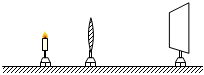$\text{A.}$ 一直变小 $\text{B.}$ 一直变大 $\text{C.}$ 先变大后变小 $\text{D.}$ 先变小后变大